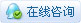# [项目] Wii Nunchuck手柄控制的6自由度机械臂

 机械臂、机械手相信大家都不陌生了，医院里的手术机械手、工厂的里的工业机械手，虽然大家从电视里或现场见过，但其庞大的身躯和高额的售价，使我们望而却步，如今一款小型廉价的6自由度机械臂，能圆大家一个玩机械手的梦！    DFRA6DOF（Robotic Arm 6 Degrees of Freedom）6自由度机械臂由6个微型伺服电机（舵机）组成，分别对应于臂、肘、腕（2个自由度）、张合5个关节和1个旋转底座，每个关节可在一定范围内运动，底座可以实现左右90度旋转。通过上位机控制软件给舵机控制器发送控制指令，从而实现机械臂在空间中的精确作业，任务完成情况可通过在机械臂上安装摄像头反馈图像。机械臂也可安装到移动平台上，可完成远程无线可视作业，适用于对人体有害的场所。关节部分杯士轴承的安装螺丝均使用圆帽螺母，防止刮伤您的手，人性化设计确保您能尽情享受那份DIY的乐趣！一、6自由度机械臂的安装所有舵机在使用前，都必须使用舵机控制器将角度调整到90度。或者通过对Arduino编程控制这个舵机旋转到90度再调整舵盘安装。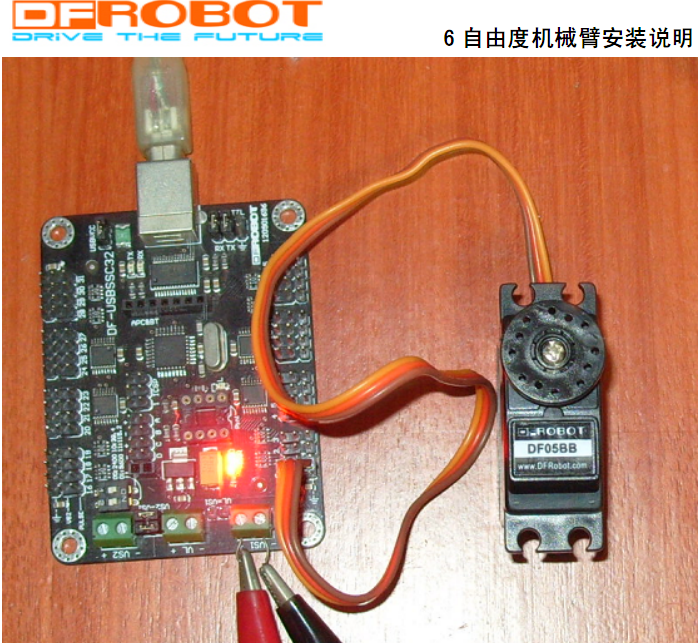使码盘四个圆孔成正十字。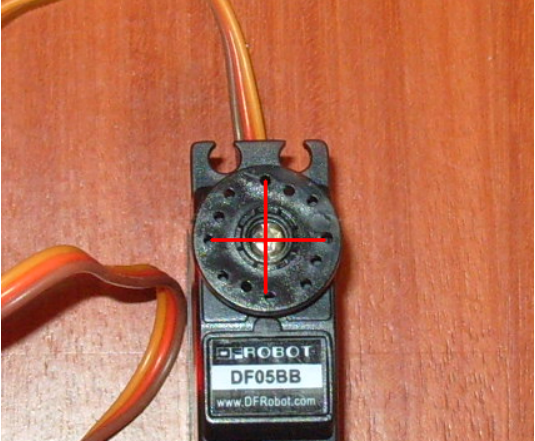将标有1号的旋转盘找出来，在其下面安装四个M3*10的单头铜柱，上面用圆帽螺母拧好。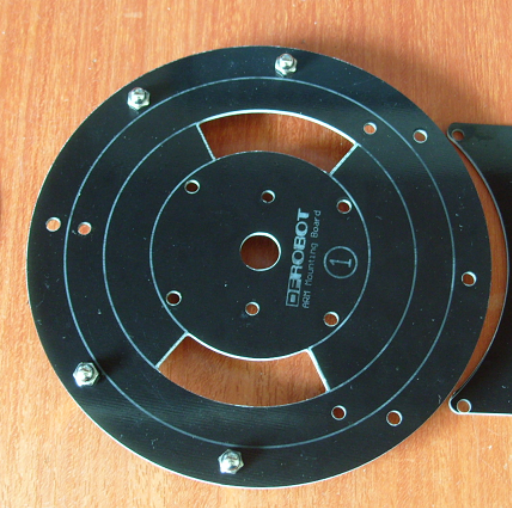接着在1号盘上面的对应位置安装5个M3*30的单头铜柱。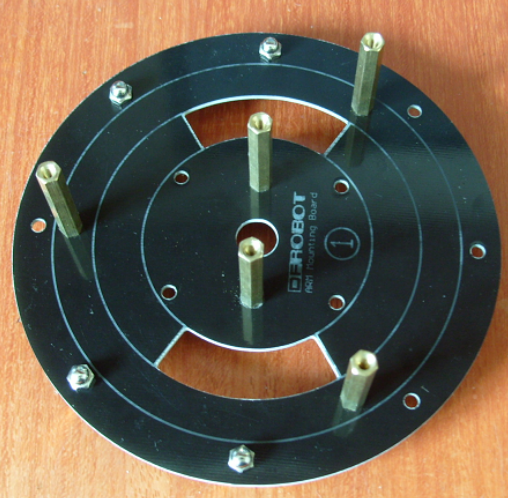·将标有4号的旋转盘找出来，在其下面对应位置安装4个M3*10的单头铜柱，上面用圆帽螺母固定好。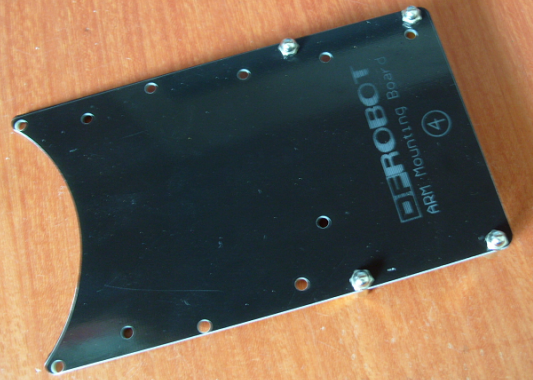将4号盘放在1号盘对应位置，用2个M3*10的单头铜柱固定好。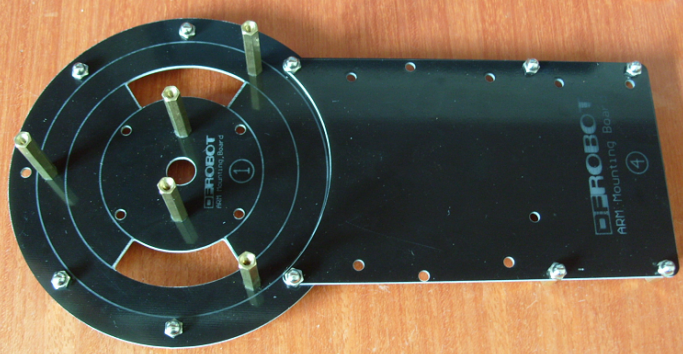将2号盘和一只归位好的DF05BB舵机准备好。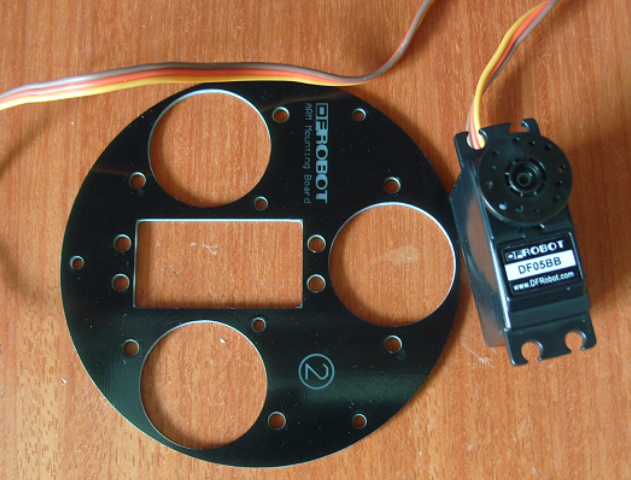将舵机按照如图位置安装在2号盘上。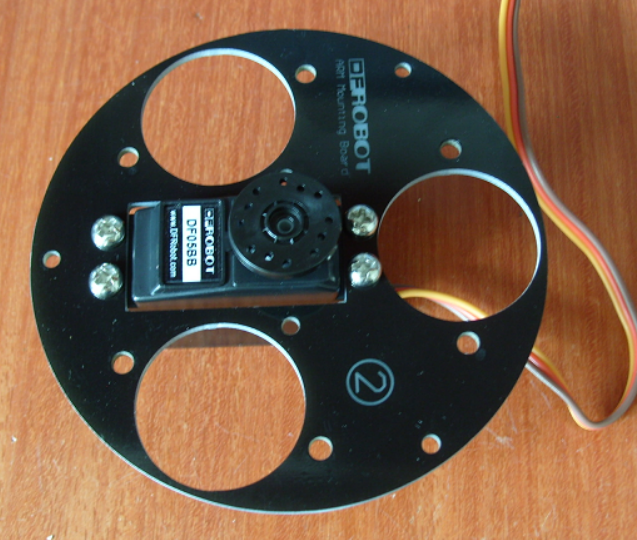在2号盘上安装3个万向轮。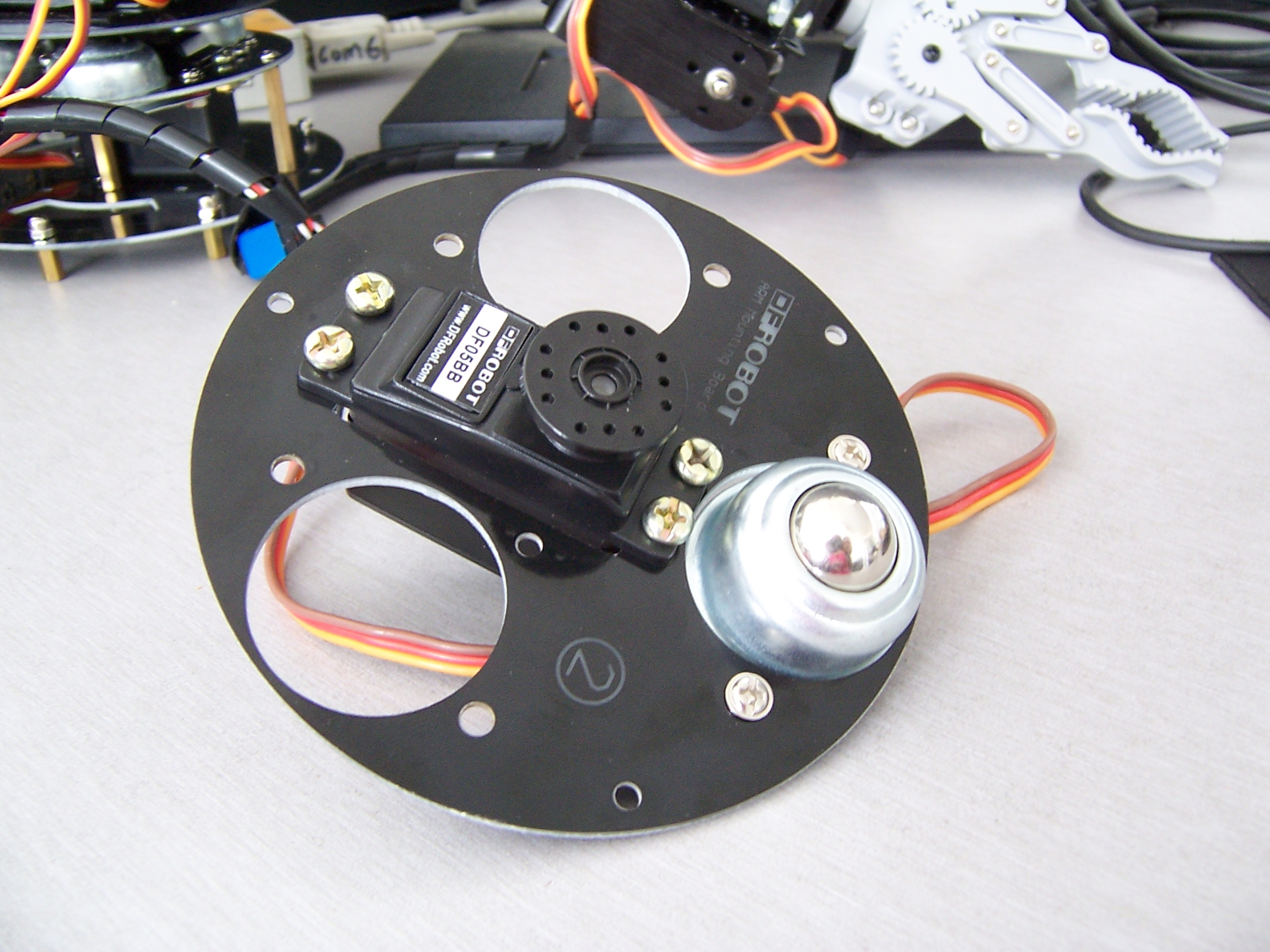详细制作过程文档及其实例代码见附件：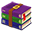Robot_Arm.zip (45.26 MB, 下载次数: 15754) 2013-7-30 10:51 上传 点击文件名下载附件 源代码：[mw_shl_code=applescript,true]#include #include #include #include "Wire.h" #include "WiiChuck.h" //定义舵机 Servo servo1; Servo servo2; Servo servo3; Servo servo4; Servo servo5; Servo servo6; WiiChuck wii = WiiChuck(); int x,y,xa,xa1=2,xa2,xa3,xa4,ya,ya1,ya2,ya3,ya4,c,z;//定义变量 void act_init()//初始化动作子函数 {   servo1.write(90);   servo2.write(70);   servo3.write(30);   servo4.write(90);   servo5.write(90);   servo6.write(30); } void setup() {   //定义舵机控制口   servo1.attach(8);   servo2.attach(9);   servo3.attach(10);   servo4.attach(11);   servo5.attach(12);   servo6.attach(13);   act_init();//初始化   wii.initWithPower();//初始化电源引脚 } void loop() {   while(1)   {     if(true==wii.read())     {       //读取手柄的值      //读取手柄的值       x=wii.getJoyAxisX();//摇杆x轴的值       y=wii.getJoyAxisY();//摇杆y轴的值       xa1=wii.getAccelAxisX();//手柄x方向摆动的值       ya1=wii.getAccelAxisY();//手柄y方向摆动的值       c=wii.getButtonC();//手柄按键c的值       z=wii.getButtonZ();//手柄按键z的值       delay(30);       x=wii.getJoyAxisX();//摇杆x轴的值       y=wii.getJoyAxisY();//摇杆y轴的值       xa2=wii.getAccelAxisX();//手柄x方向摆动的值       ya2=wii.getAccelAxisY();//手柄y方向摆动的值       c=wii.getButtonC();//手柄按键c的值       z=wii.getButtonZ();//手柄按键z的值       delay(30);       x=wii.getJoyAxisX();//摇杆x轴的值       y=wii.getJoyAxisY();//摇杆y轴的值       xa3=wii.getAccelAxisX();//手柄x方向摆动的值       ya3=wii.getAccelAxisY();//手柄y方向摆动的值       c=wii.getButtonC();//手柄按键c的值       z=wii.getButtonZ();//手柄按键z的值       delay(30);       x=wii.getJoyAxisX();//摇杆x轴的值       y=wii.getJoyAxisY();//摇杆y轴的值       xa4=wii.getAccelAxisX();//手柄x方向摆动的值       ya4=wii.getAccelAxisY();//手柄y方向摆动的值       c=wii.getButtonC();//手柄按键c的值       z=wii.getButtonZ();//手柄按键z的值       delay(30);       xa=(xa1+xa2+xa3+xa4)/4;//取均值       xa=xa-40;//为了让90成为中点值       ya=(ya1+ya2+ya3+ya4)/4;//取平均值       ya=ya-40;     }     if((x==130||x==131)&&y==255&&z==1)//摇杆位置为向前     {       if(xa<30)//限制最小值         xa=30;       if(xa>150)//限制最大值         xa=150;       servo1.write(xa);//给舵机旋转的度数       delay(10);     }     else if((x==130||x==131)&&y==0)//摇杆位置向后     {       if(ya<30)         ya=30;       if(ya>150)         ya=150;       servo2.write(ya);//给舵机旋转的度数       delay(10);     }     else if(x==0&&(y==130||y==129))//摇杆的位置向左     {       ya=175-ya;//为了让手柄在Y轴向前的时候，舵机3也向前       if(ya<20)         ya=20;       if(ya>150)         ya=150;       servo3.write(ya);//给舵机旋转的度数       delay(10);     }     else if(x==255&&(y==130||y==129))//摇杆的位置向右     {       ya=175-ya;       if(ya<30)         ya=30;       if(ya>80)         ya=80;       servo4.write(ya);//给舵机旋转的度数       delay(10);     }     else if(c==0)//手柄按键c按下     {       if(xa<30)         xa=30;       if(xa>150)         xa=150;       servo5.write(xa);//给舵机旋转的度数       delay(10);     }     else if(z==0)//手柄按键z按下     {       if(ya<40)         ya=40;       if(ya>110)         ya=110;       servo6.write(ya);//给舵机旋转的度数       delay(10);     }   } }[/mw_shl_code]
 您需要登录后才可以回帖 登录 | 立即注册 本版积分规则 回帖并转播 回帖后跳转到最后一页

[[wsData.name]]

#### 硬件清单

• [[d.name]]#### 楼主的其它帖子

© 2013-2021 Comsenz Inc. Powered by Discuz! X3.4 Licensed